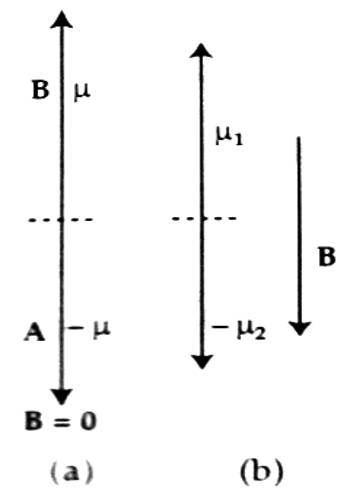Physics

# Diamagnetism of Diamagnetic MaterialsDiamagnetism of Diamagnetic Materials

The materials which have net magnetic moments i.e., those materials which reveal para and ferromagnetism, the diamagnetism in those materials becomes overshadowed due to its weak value. So, Diamagnetism is a quantum mechanical effect that occurs in all materials; when it is the only contribution to the magnetism, the material is called diamagnetic.

When a diamagnetic substance is placed in a magnetic field B, a change in orbital motion of the atoms occurs. That means the angular velocity increases or decreases due to the influence of the magnetic field increase or decrease depends on the direction of rotation. In short, it can be said that that material which, when placed in a magnetic field acquire a small amount of magnetism opposite to the direction of the inducing magnetic field are called diamagnetic materials. Examples – copper, silver, zinc, bismuth, lead, glass, marble, helium, water, argon, sodium chloride etc.

Due to the change of angular velocity, the orbital magnetic moment of the electrons also changes. If the angular velocity decreases, the magnetic moment also decreases. On the other hand if the angular velocity increases, the magnetic moment also increases. So, it is seen that as the magnetic field B is applied on a diamagnetic substance, a magnetic moment is induced and its direction is opposite to the applied magnetic field B; consequently, there is repulsion. This is the reason that a diamagnetic material is repelled when it is brought nearer to a strong magnet.In the figure, magnetic moments of two electrons A and B are shown. When the external magnetic field B = 0, then the magnetic moments of those two electrons cancel each other [Fig. (a)] but as the magnetic field is applied magnetic moment does not disappear; a net magnetic moment results as shown in [fig. (b)]. The direction of this net moment is opposite to the applied magnetic field B.